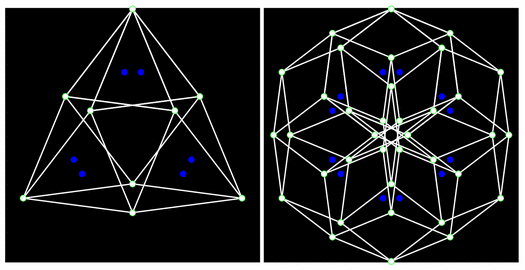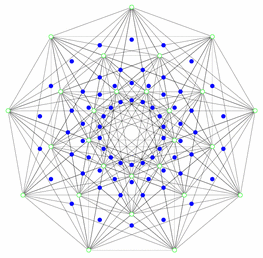# pst-cox

## Coxeter Polytopes

PST-Cox is a LaTeX library for drawing 2D projections of regular complex polytopes. The regular complex polytopes are hyperplans arrangements with some constraints whose automorphisms groups are generated by pseudo-reflections (complex reflections). They generalize the classical Platon solids.

In the following example, the complex polytopes β32 and β62 are drawn. Their edges are respectively triangular and hexagonal.The regular complex polytope are related to the study of the roots systems and are used in Mathematics as well as in Physics. For example, some degenerate cases of the ternary trilinear forms admits normal form which are the vertices of an Hessian polytope (regular complex polytope in dimension 3).Its group of automorphisms is a subgroup of order 648 of the Mashke group which has been considered in the study of the 27 lines of a general cubic plane in P3. This polytope have been considered by one of the author of the Library in collaboration with E. Briand, J.-Y. Thibon and F. Verstraete in the study of the normal form of the three-qubit systems.

Auteur : Jean-Gabriel Luque